# Test methodologies Functional Black box inspects specified behavior

• Slides: 20Test methodologies • Functional (Black box) inspects specified behavior • Structural (White box) inspects programmed behavior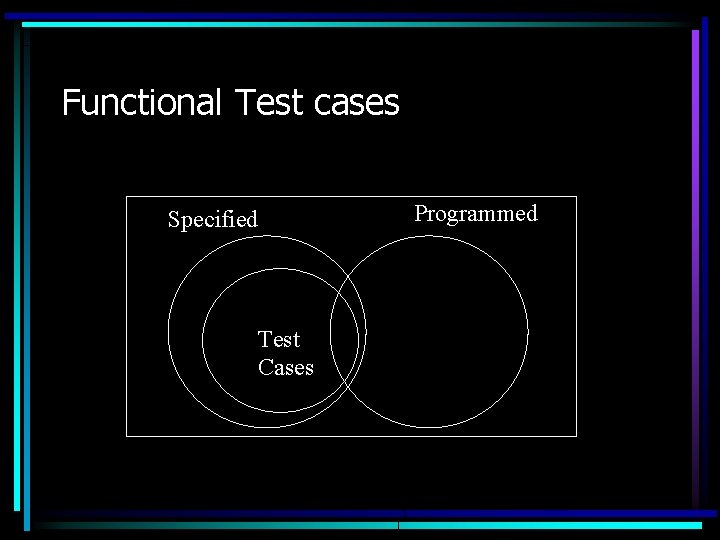Functional Test cases Specified Test Cases Programmed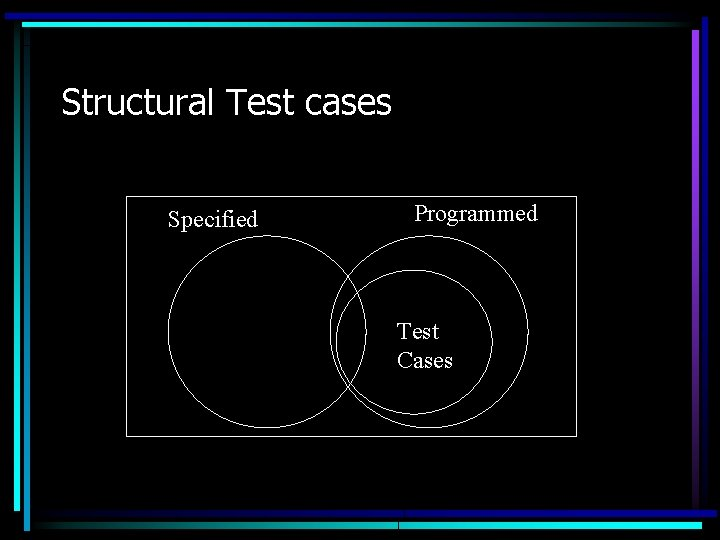Structural Test cases Specified Programmed Test Cases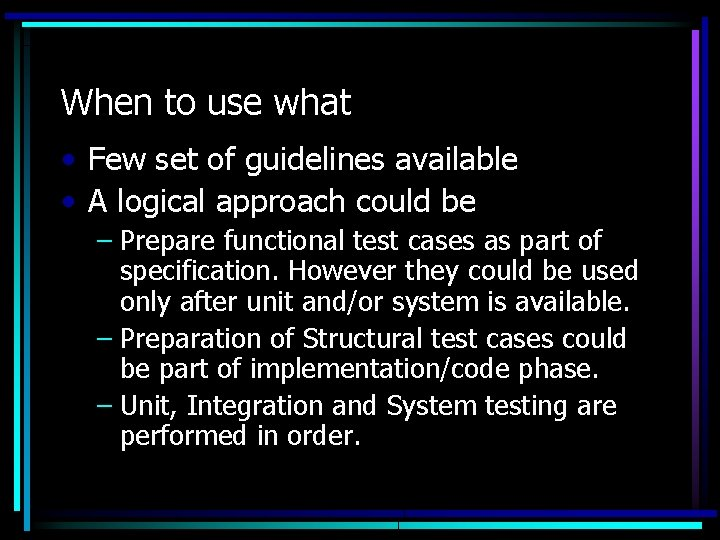When to use what • Few set of guidelines available • A logical approach could be – Prepare functional test cases as part of specification. However they could be used only after unit and/or system is available. – Preparation of Structural test cases could be part of implementation/code phase. – Unit, Integration and System testing are performed in order.Unit testing – essence • Applicable to modular design – Unit testing inspects individual modules • Locate error in smaller region – In an integrated system, it may not be easier to determine which module has caused fault – Reduces debugging efforts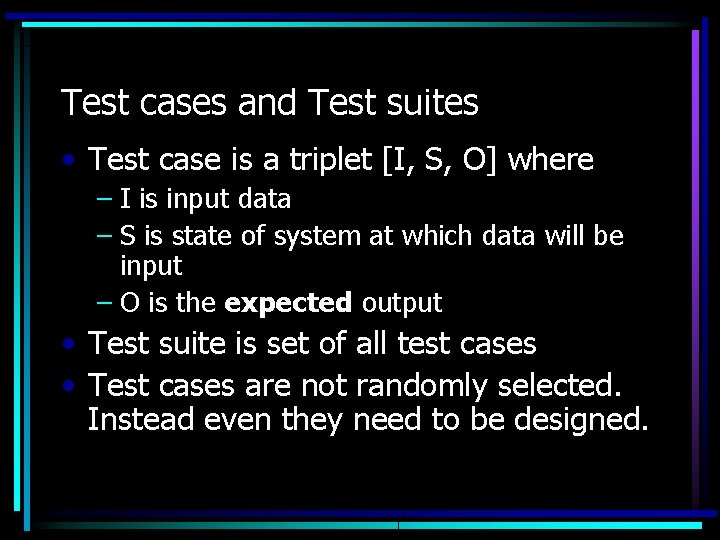Test cases and Test suites • Test case is a triplet [I, S, O] where – I is input data – S is state of system at which data will be input – O is the expected output • Test suite is set of all test cases • Test cases are not randomly selected. Instead even they need to be designed.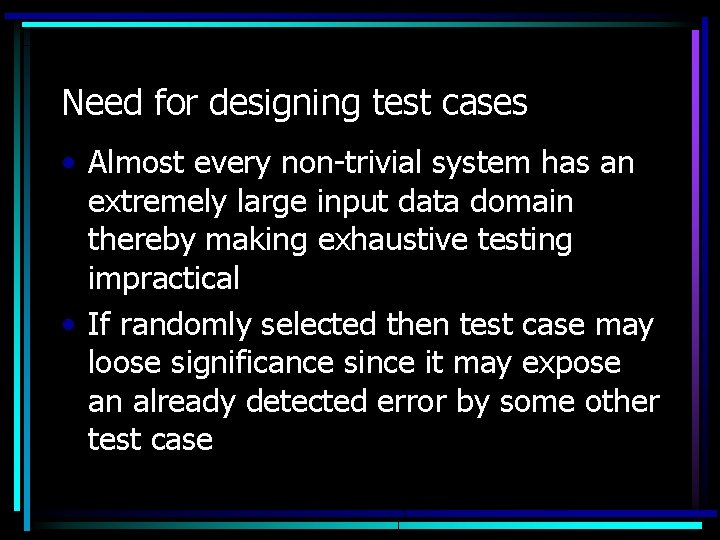Need for designing test cases • Almost every non-trivial system has an extremely large input data domain thereby making exhaustive testing impractical • If randomly selected then test case may loose significance since it may expose an already detected error by some other test case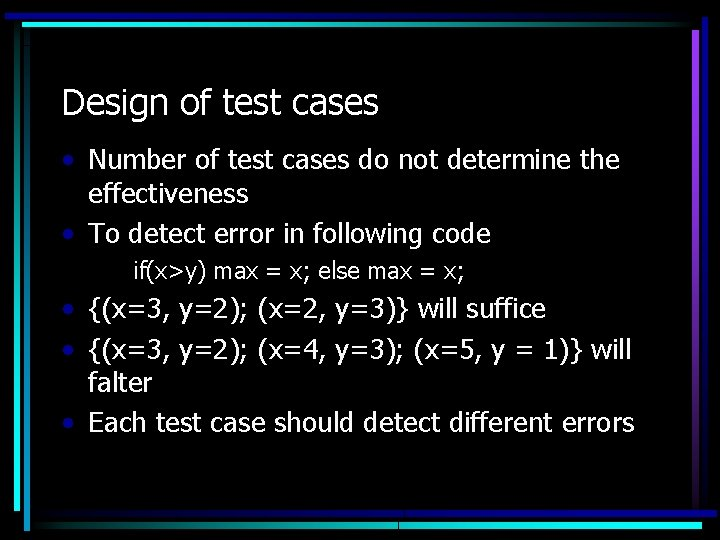Design of test cases • Number of test cases do not determine the effectiveness • To detect error in following code if(x>y) max = x; else max = x; • {(x=3, y=2); (x=2, y=3)} will suffice • {(x=3, y=2); (x=4, y=3); (x=5, y = 1)} will falter • Each test case should detect different errors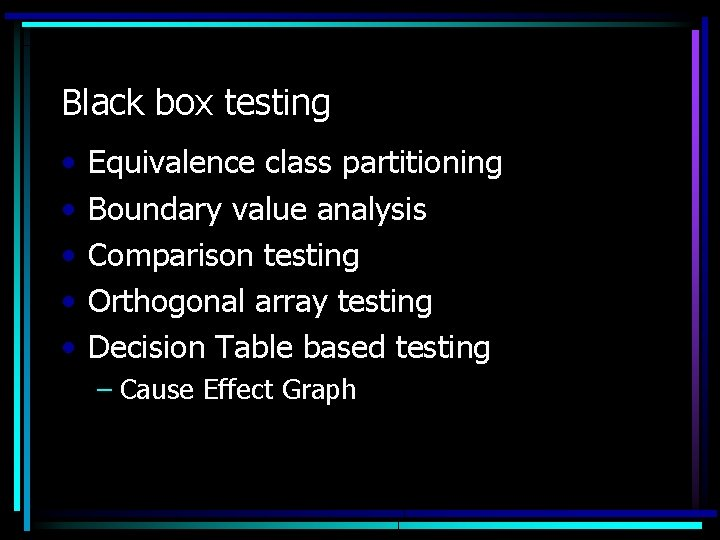Black box testing • • • Equivalence class partitioning Boundary value analysis Comparison testing Orthogonal array testing Decision Table based testing – Cause Effect Graph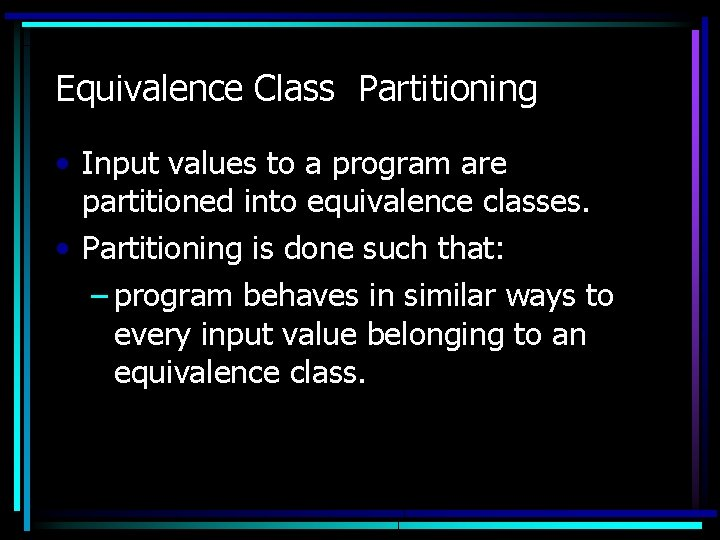Equivalence Class Partitioning • Input values to a program are partitioned into equivalence classes. • Partitioning is done such that: – program behaves in similar ways to every input value belonging to an equivalence class.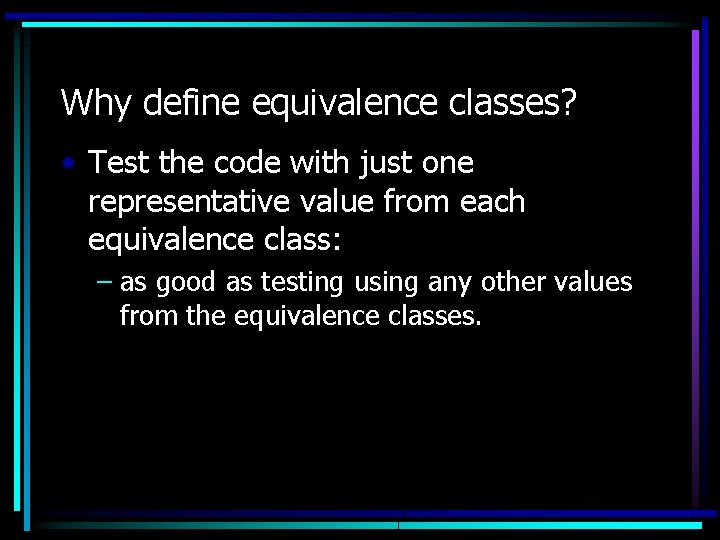Why define equivalence classes? • Test the code with just one representative value from each equivalence class: – as good as testing using any other values from the equivalence classes.Equivalence Class Partitioning • How do you determine the equivalence classes? – examine the input data. – few general guidelines for determining the equivalence classes can be given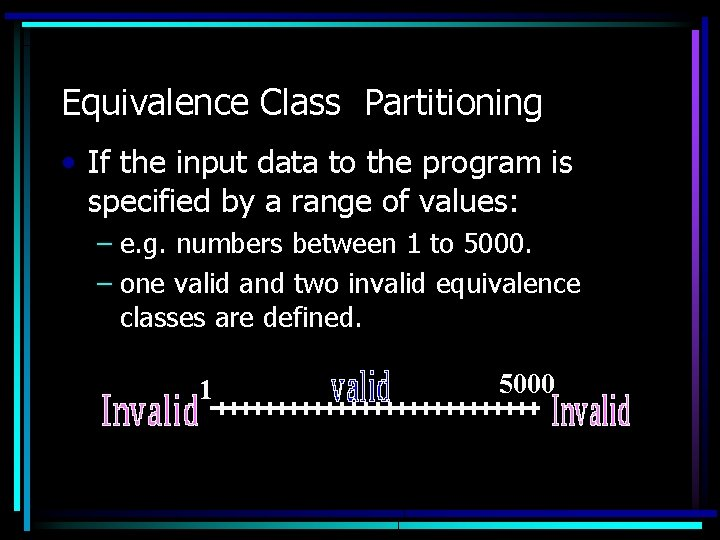Equivalence Class Partitioning • If the input data to the program is specified by a range of values: – e. g. numbers between 1 to 5000. – one valid and two invalid equivalence classes are defined. 1 5000Equivalence Class Partitioning • If input is an enumerated set of values: – e. g. {a, b, c} – one equivalence class for valid input values – another equivalence class for invalid input values should be defined.Example • A program reads an input value in the range of 1 and 5000: – computes the square root of the input number SQRTExample (cont. ) • There are three equivalence classes: – the set of negative integers, – set of integers in the range of 1 and 5000, – integers larger than 5000. 1 5000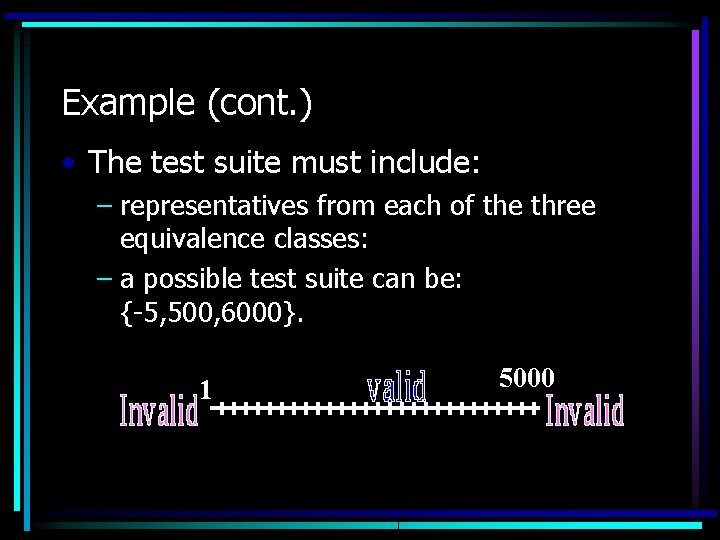Example (cont. ) • The test suite must include: – representatives from each of the three equivalence classes: – a possible test suite can be: {-5, 500, 6000}. 1 5000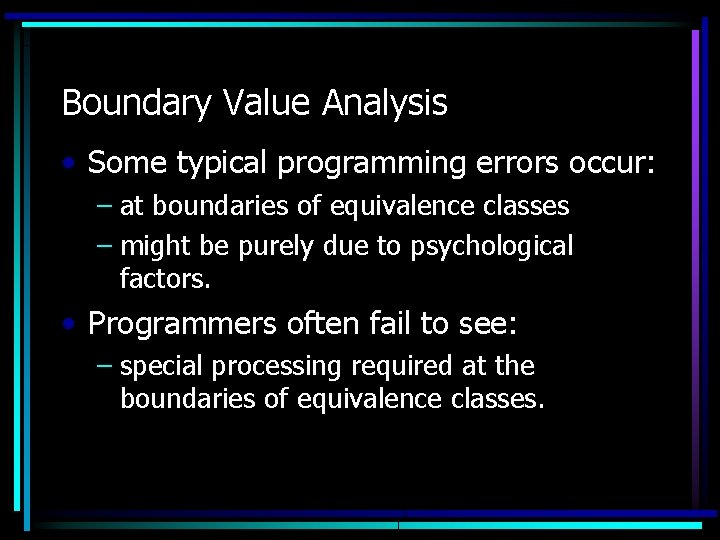Boundary Value Analysis • Some typical programming errors occur: – at boundaries of equivalence classes – might be purely due to psychological factors. • Programmers often fail to see: – special processing required at the boundaries of equivalence classes.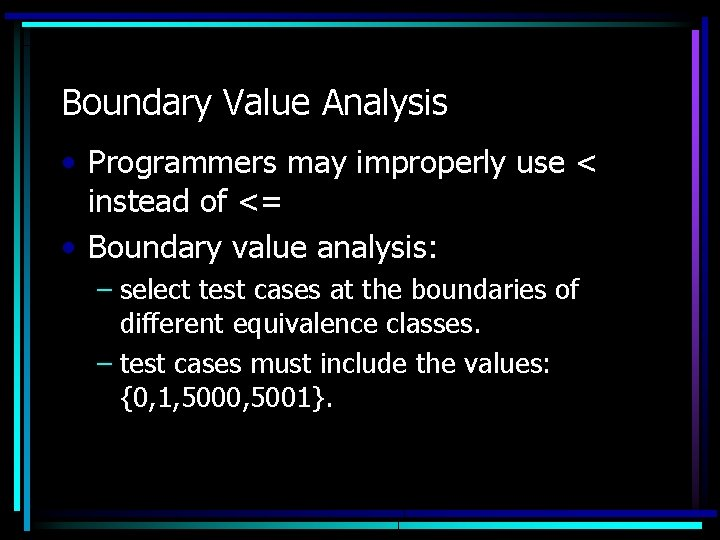Boundary Value Analysis • Programmers may improperly use < instead of <= • Boundary value analysis: – select test cases at the boundaries of different equivalence classes. – test cases must include the values: {0, 1, 5000, 5001}.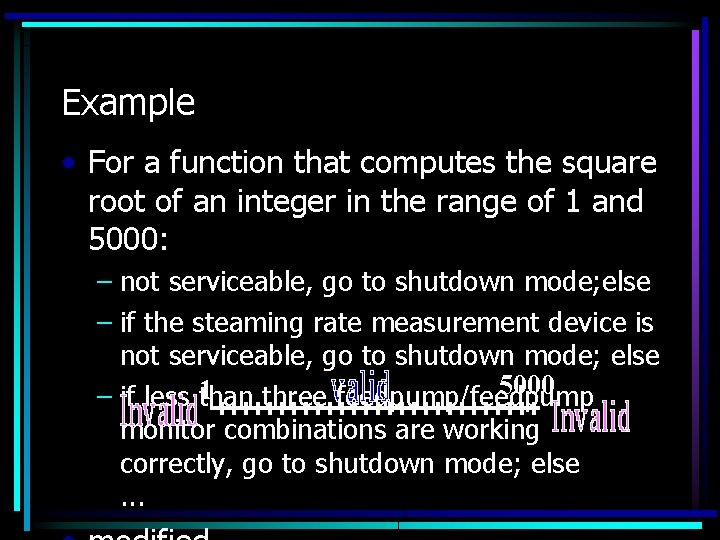Example • For a function that computes the square root of an integer in the range of 1 and 5000: – not serviceable, go to shutdown mode; else – if the steaming rate measurement device is not serviceable, go to shutdown mode; else 5000 1 – if less than three feedpump/feedpump monitor combinations are working correctly, go to shutdown mode; else. . .